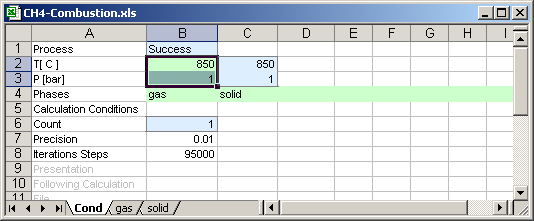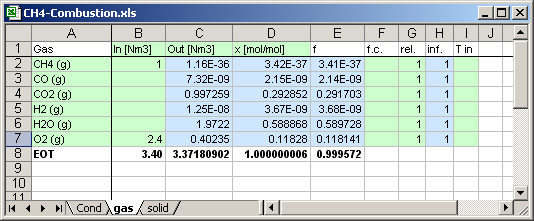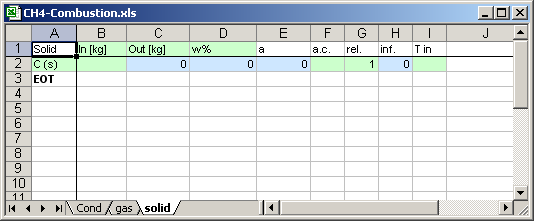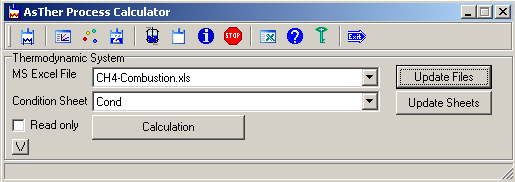AsTher Thermodynamic Database and Applications

AsTher Process Calculator for MS Excel

8.2 CH4-Combustion, Simple calculation using AsTher Process Calculator for MS Excel

This example shows a simple calculation.

In the MS Excel file, first sheet named Cond contains the calculation conditions
The temperature and pressure of the thermodynamic system given in the cells  B2 and B3
The thermodynamic systems contains two phases gas and solid.
The phases gas and solid defined in the sheets gas and solid.

The green coloured cells are written by user before calculation
The blue coloured cells are written by Process Calculator after the calculationThe compounds of the gas phase are given in the cells A2 to A7.
EOT in the cell A8 means the end of the table for thermodynamic system.
The rows 8 and below 8 is not used by Process calculator
Input values are given in the cell B2 for CH4 and in the cell B7 for O2.The compounds of the solid phase are given in the cells A2 .
EOT in the cell A3 means end of the table. The rows 3 and below 3 is not used by Process Calculator

The phase solid contains only one substance: carbon.
Therefore the calculation regards, that the carbon can form only as pure substance in the thermodynamic system.
No any input value is given for C.When the data in the green coloured cells are written by user, the application Process Calculator for MS Excel runs, and button Calculation is pressed, then the blue coloured cells will be written by application.